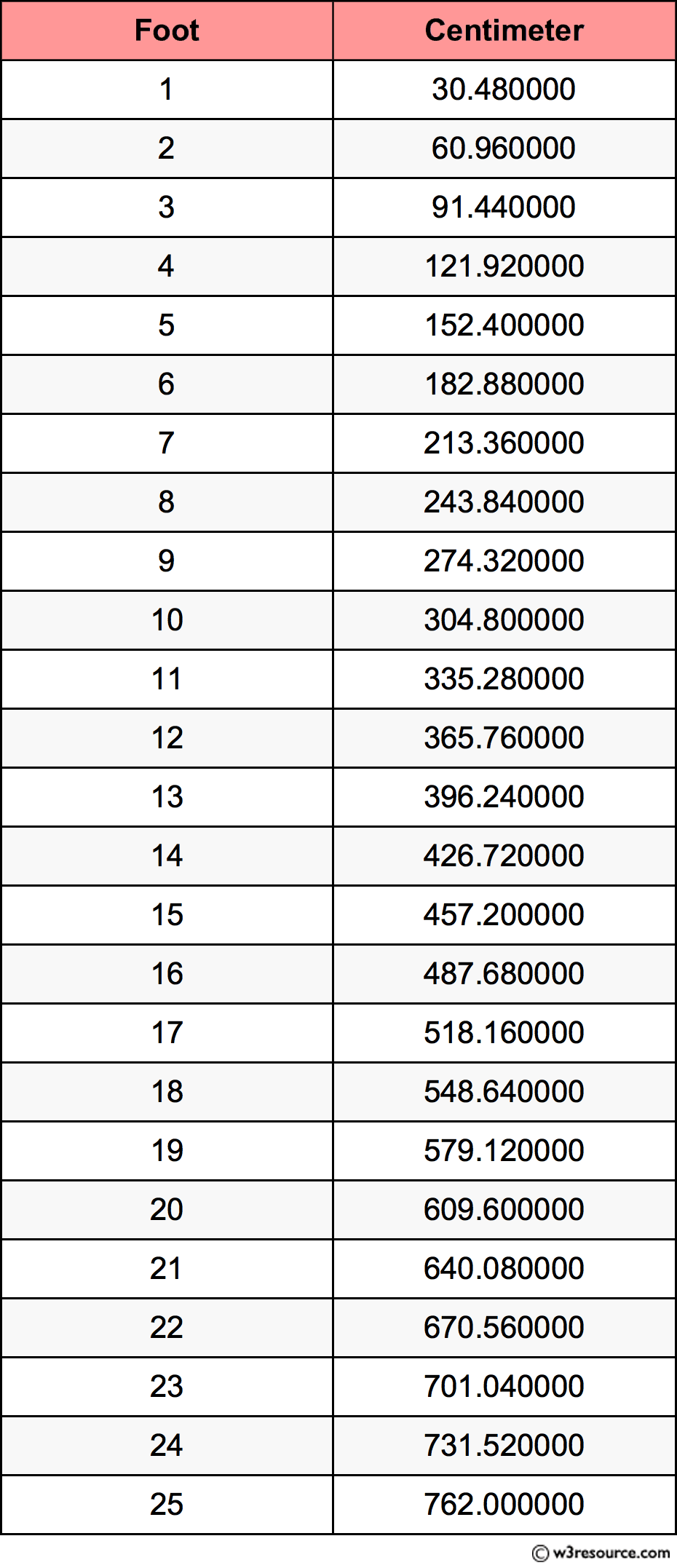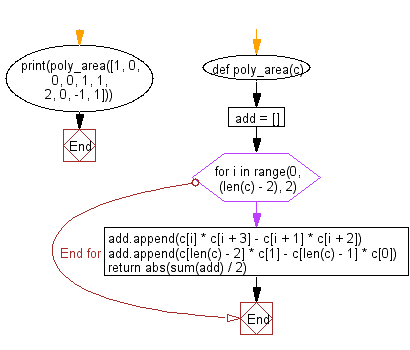﻿ Python: Convert height (in feet and inches) to centimeters - w3resource# Python: Convert height (in feet and inches) to centimeters

## Python Basic: Exercise-59 with Solution

Write a Python program to convert height (in feet and inches) to centimeters.

Pictorial Presentation:Sample Solution:-

Python Code:

``````print("Input your height: ")
h_ft = int(input("Feet: "))
h_inch = int(input("Inches: "))

h_inch += h_ft * 12
h_cm = round(h_inch * 2.54, 1)

print("Your height is : %d cm." % h_cm)
```
```

Sample Output:

```Input your height:
Feet:  5
Inches:  3
Your height is : 160 cm.
```

Flowchart:## Visualize Python code execution:

The following tool visualize what the computer is doing step-by-step as it executes the said program:

Python Code Editor:

Have another way to solve this solution? Contribute your code (and comments) through Disqus.

What is the difficulty level of this exercise?

Test your Python skills with w3resource's quiz

﻿

## Python: Tips of the Day

Unknown Arguments Using *arguments:

If your function can take in any number of arguments then add a * in front of the parameter name:

```def myfunc(*arguments):
for a in arguments:
print a
myfunc(a)
myfunc(a,b)
myfunc(a,b,c)
```